# NCERT Solutions for Class 7 Maths Chapter 8 – Comparing Quantities

Class 7 Maths NCERT Solutions Chapter 8 – Comparing Quantities comprises of the 3 Exercises

This Chapter contains the Exercises relating to the following topics , which are discussed in Chapter 8 – Comparing Quantities Class 7 NCERT book  : –

• 8.1 INTRODUCTION
• 8.2 EQUIVALENT RATIOS
• 8.3 PERCENTAGE – ANOTHER WAY OF COMPARING QUANTITIES
• 8.3.1 Meaning of Percentage
• 8.3.2 Converting Fractional Numbers to Percentage
• 8.3.3 Converting Decimals to Percentage
• 8.3.4 Converting Percentages to Fractions or Decimals
• 8.3.5 Fun with Estimation
• 8.4 USE OF PERCENTAGES
• 8.4.1 Interpreting Percentages
• 8.4.2 Converting Percentages to “How Many”
• 8.4.3 Ratios to Percents
• 8.4.4 Increase or Decrease as Per Cent
• 8.5 PRICES RELATED TO AN ITEM OR BUYING AND SELLING
• 8.5.1 Profit or Loss as a Percentage
• 8.6 CHARGE GIVEN ON BORROWED MONEY OR SIMPLE INTEREST
• 8.6.1 Interest for Multiple Years

### NCERT Solutions for Class 7 Maths Chapter 8 Exercise 8.1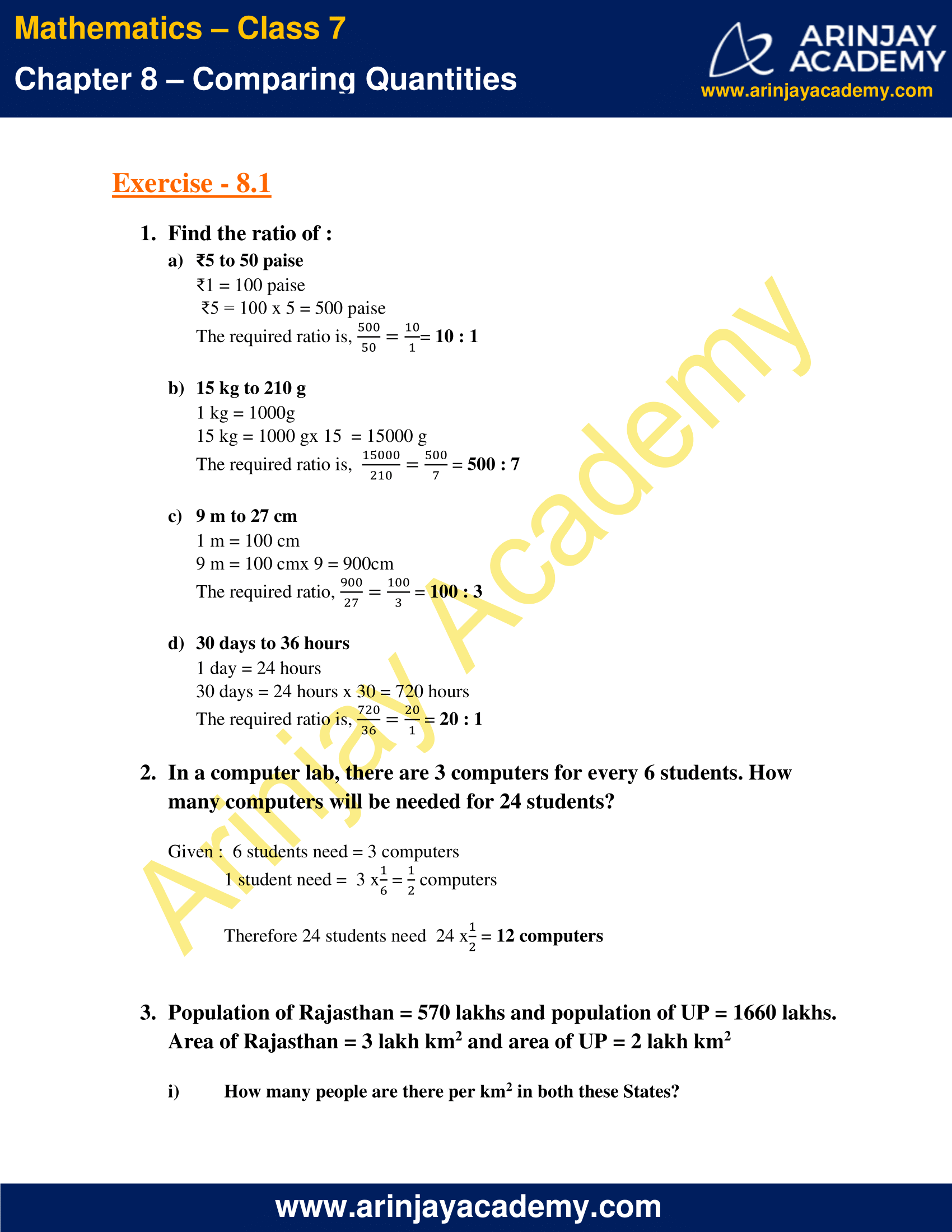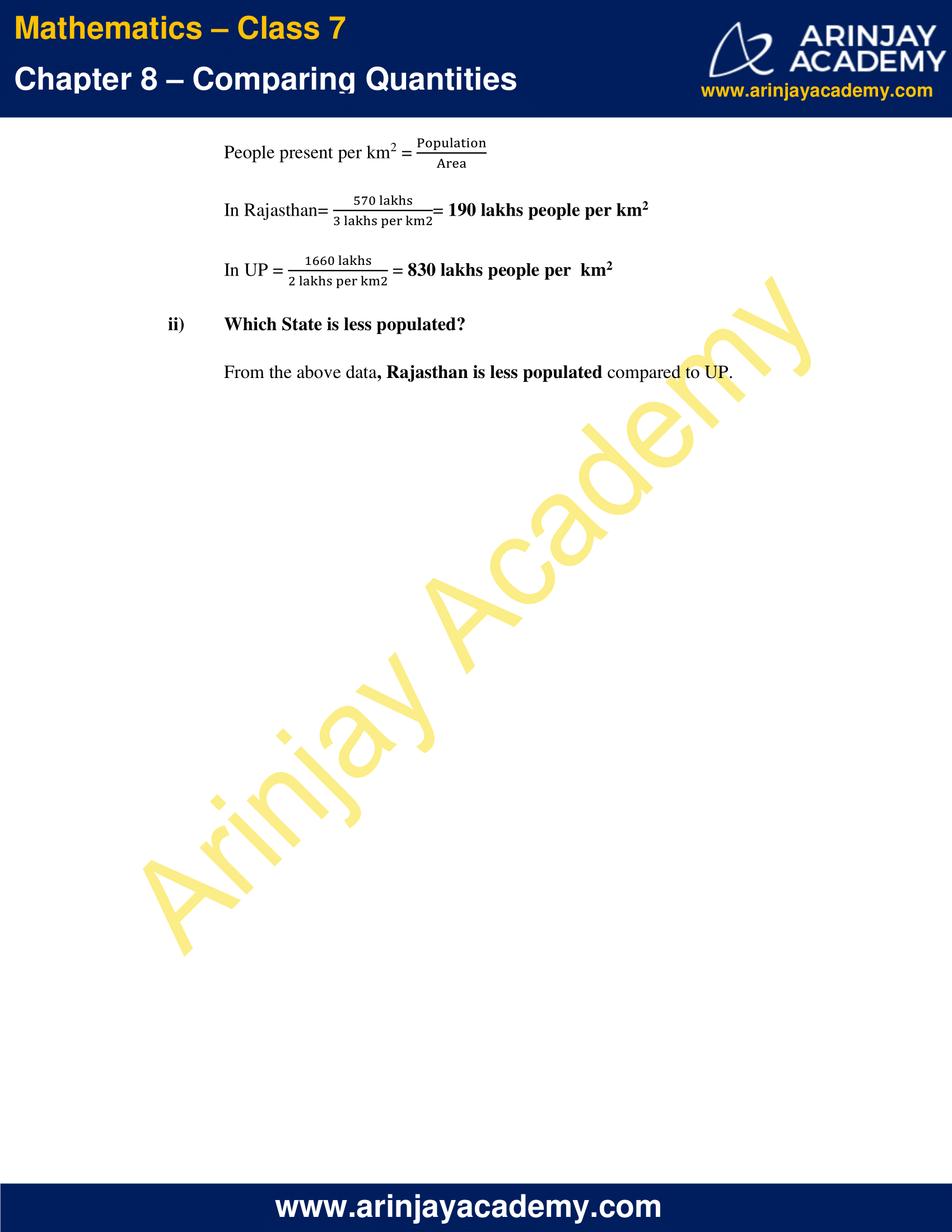### NCERT Solutions for Class 7 Maths Chapter 8 Exercise 8.2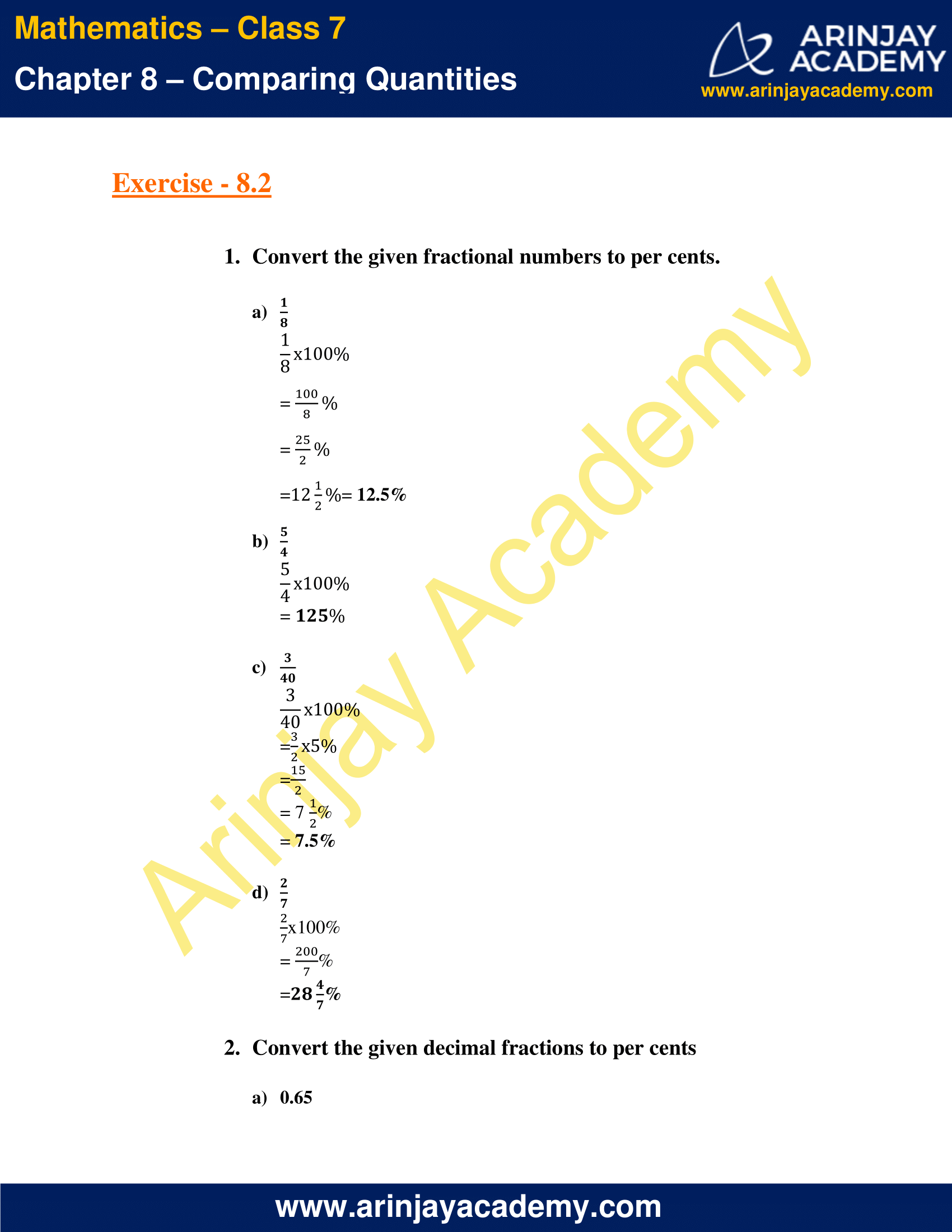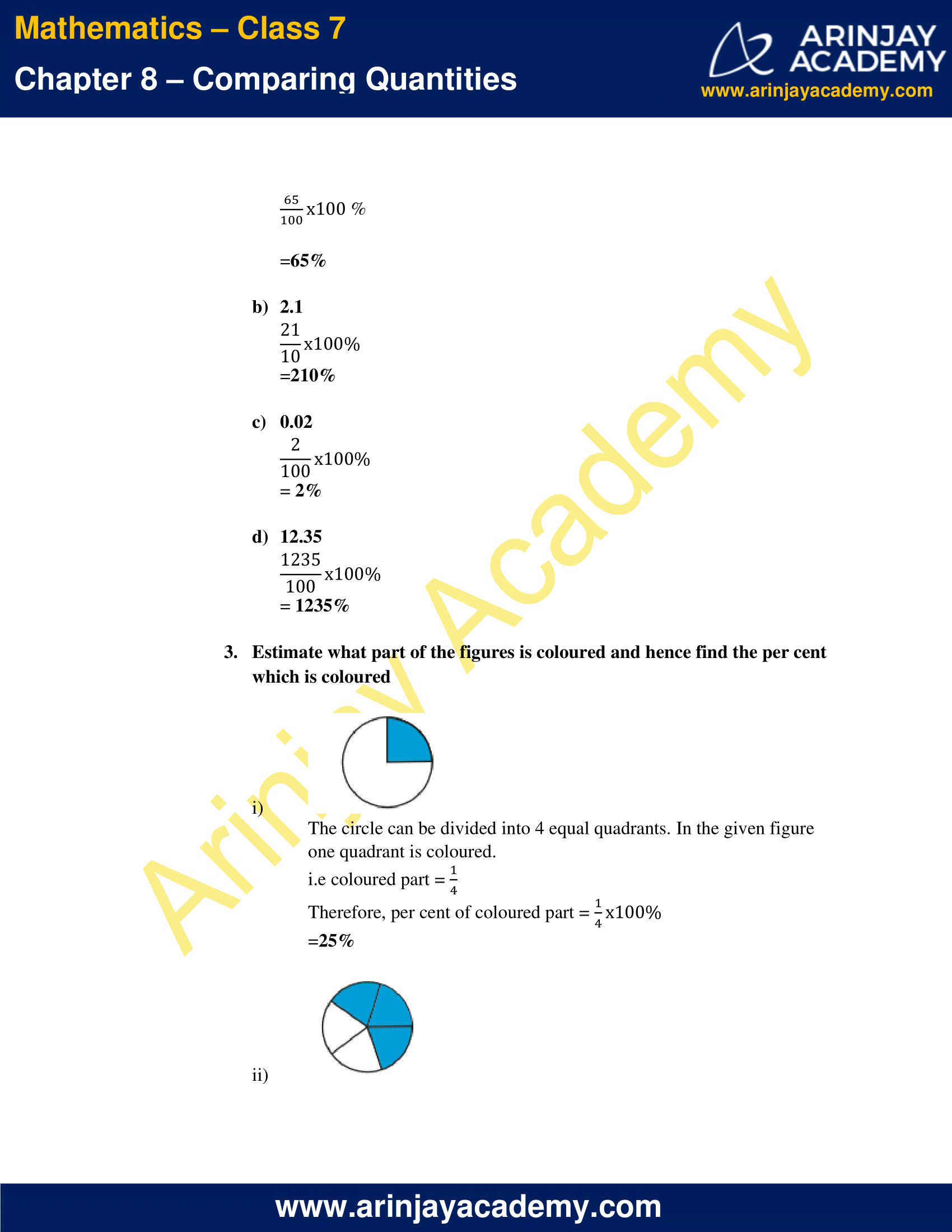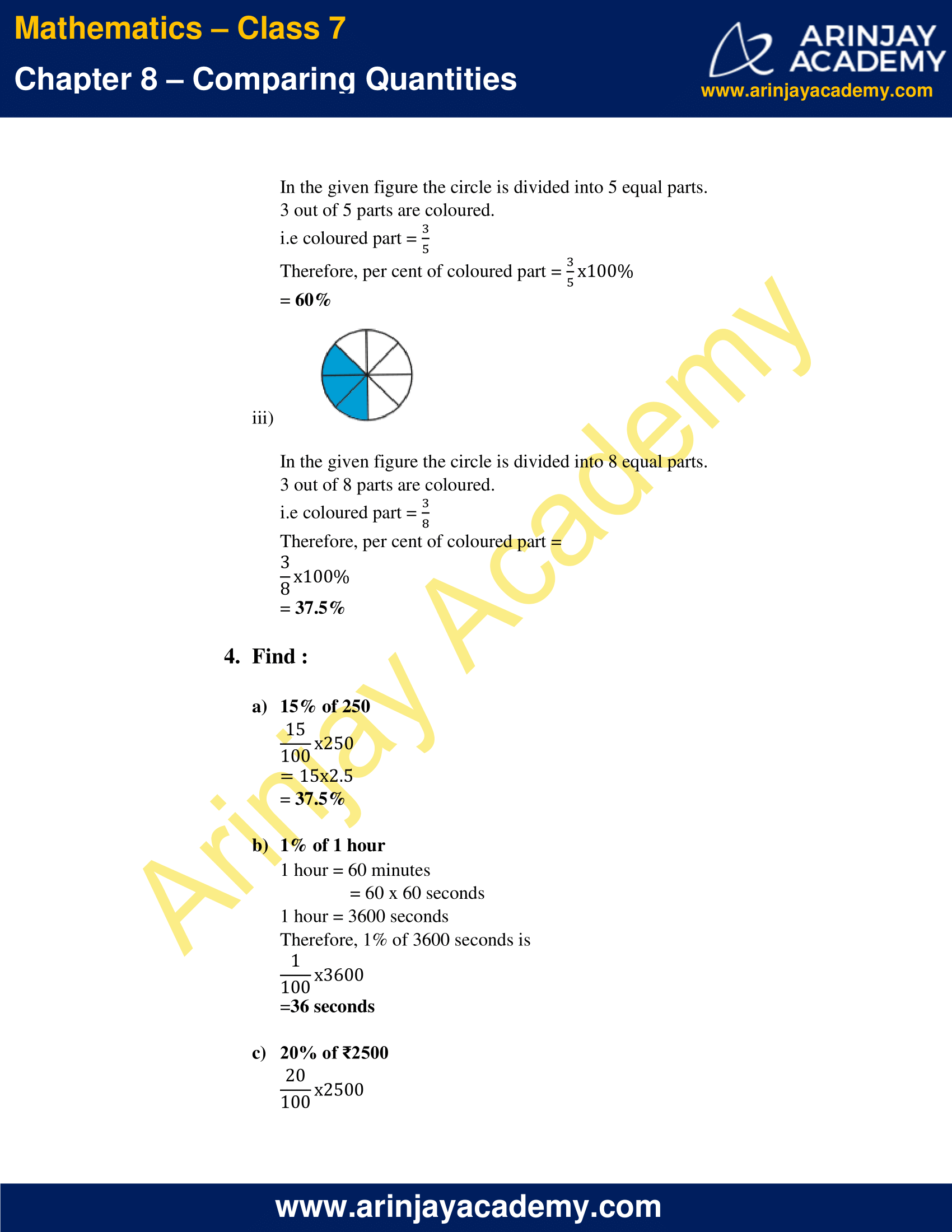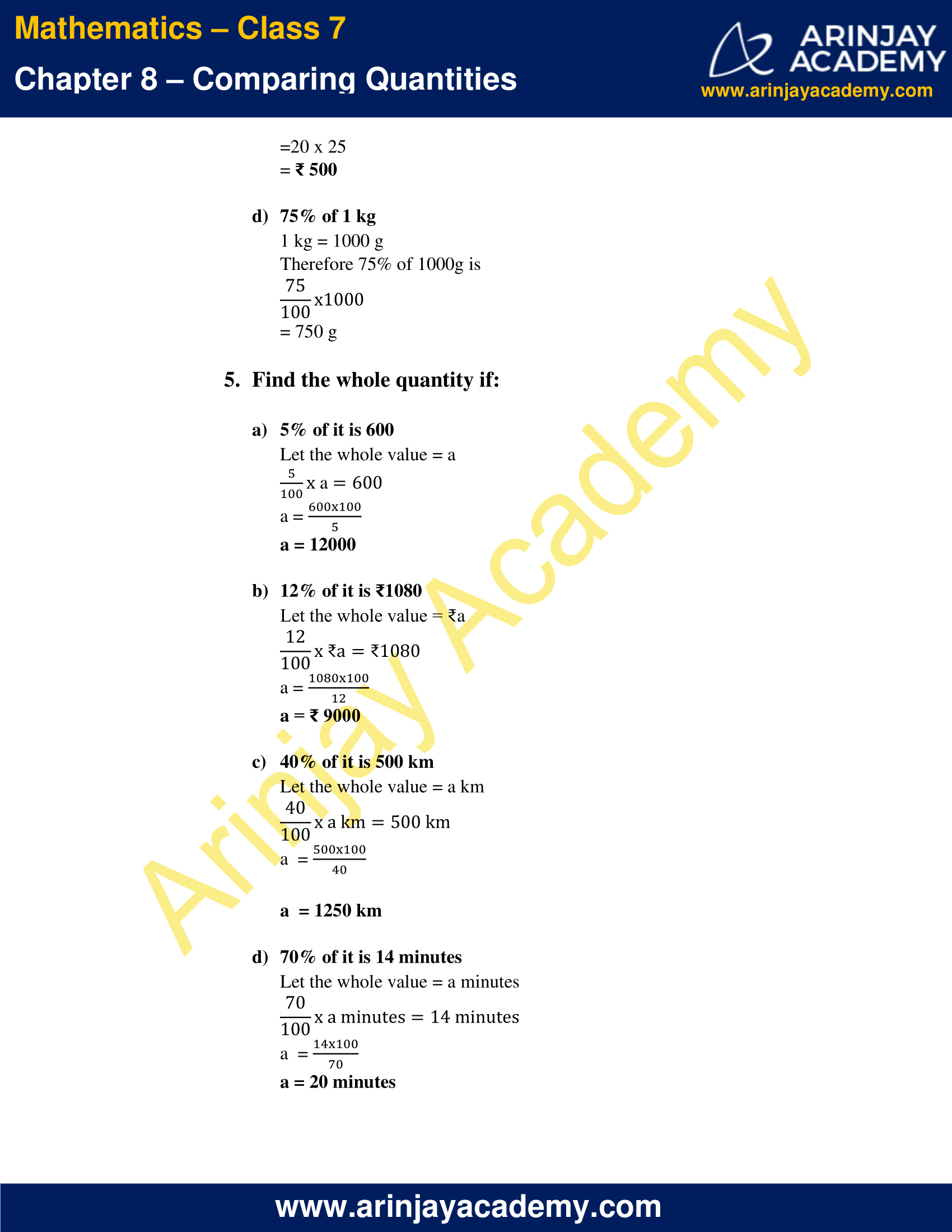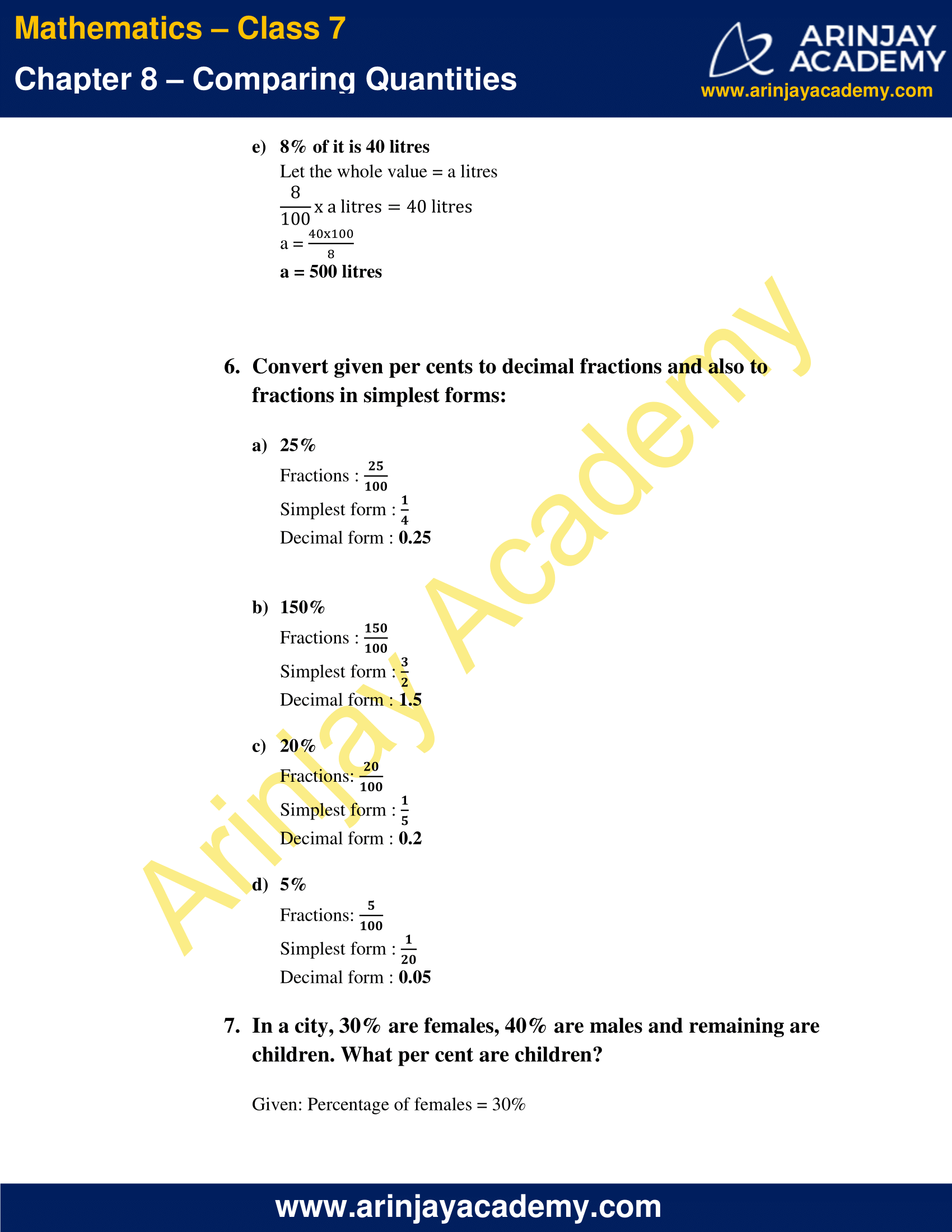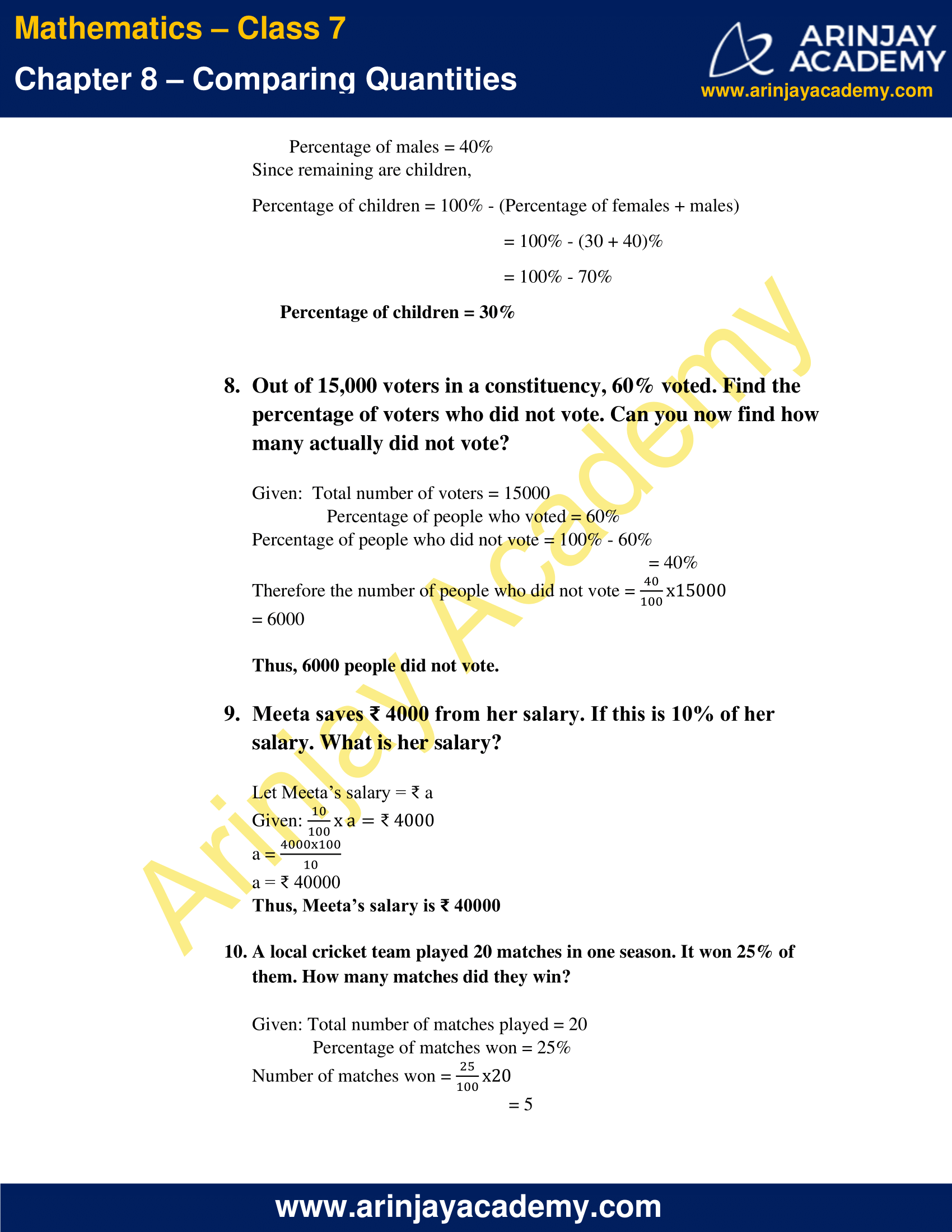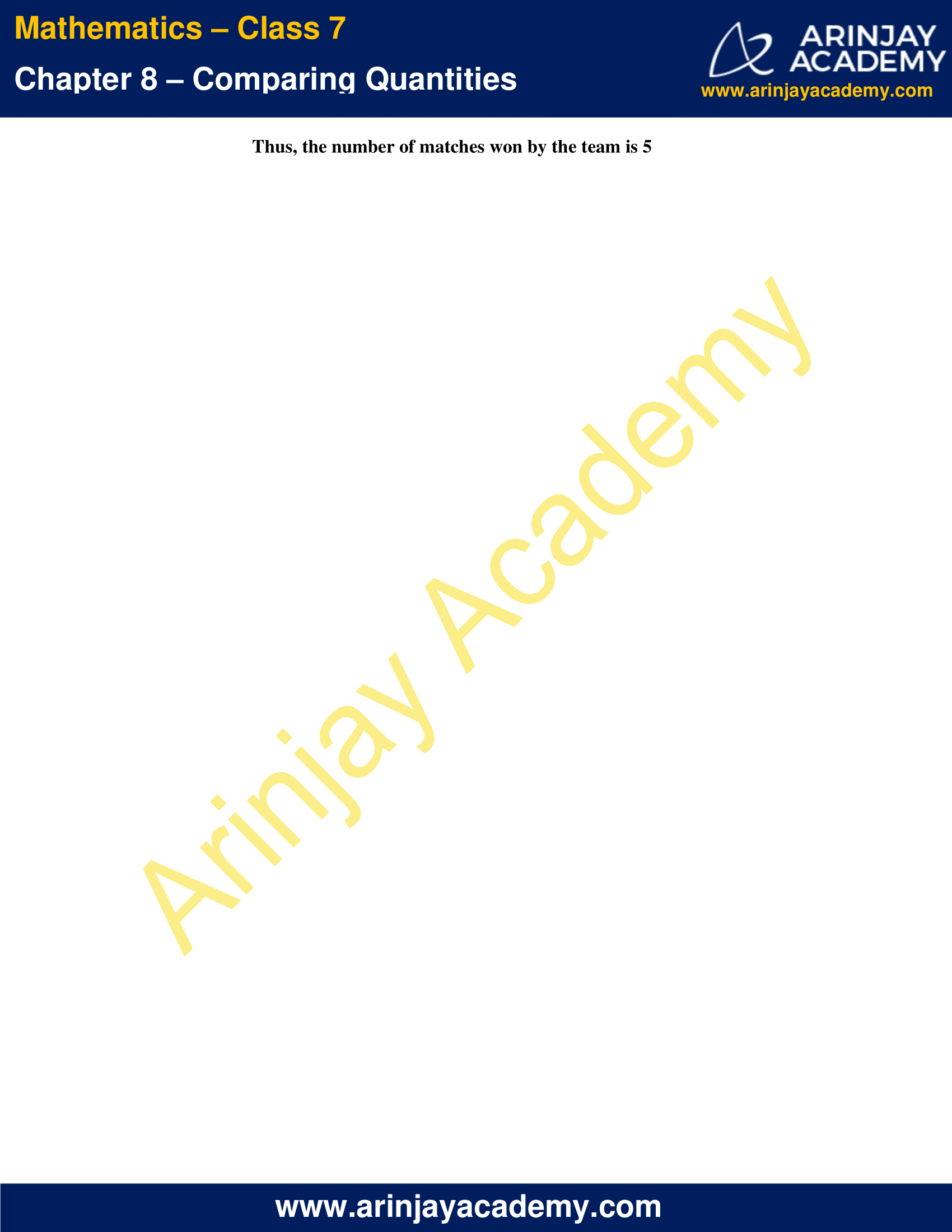### NCERT Solutions for Class 7 Maths Chapter 8 Exercise 8.3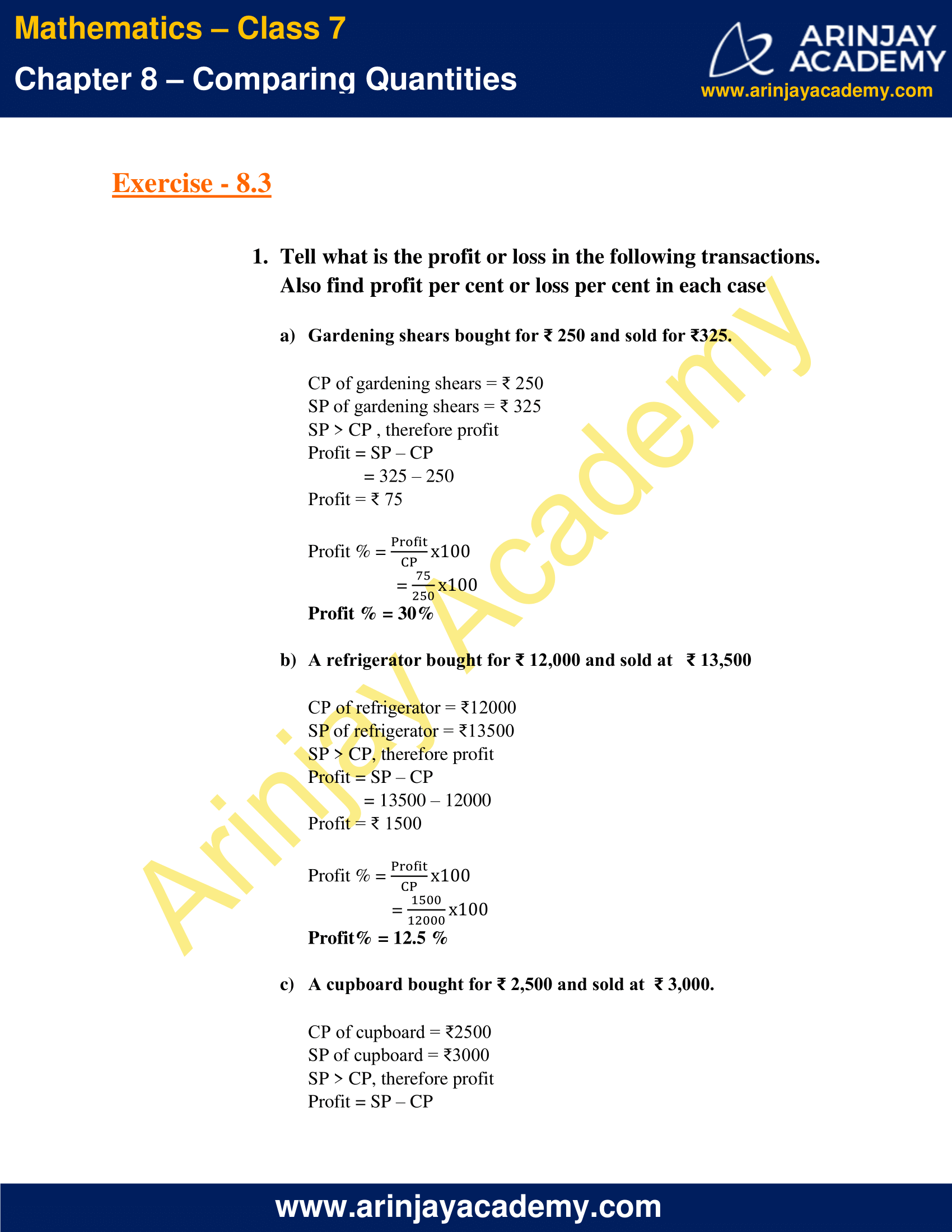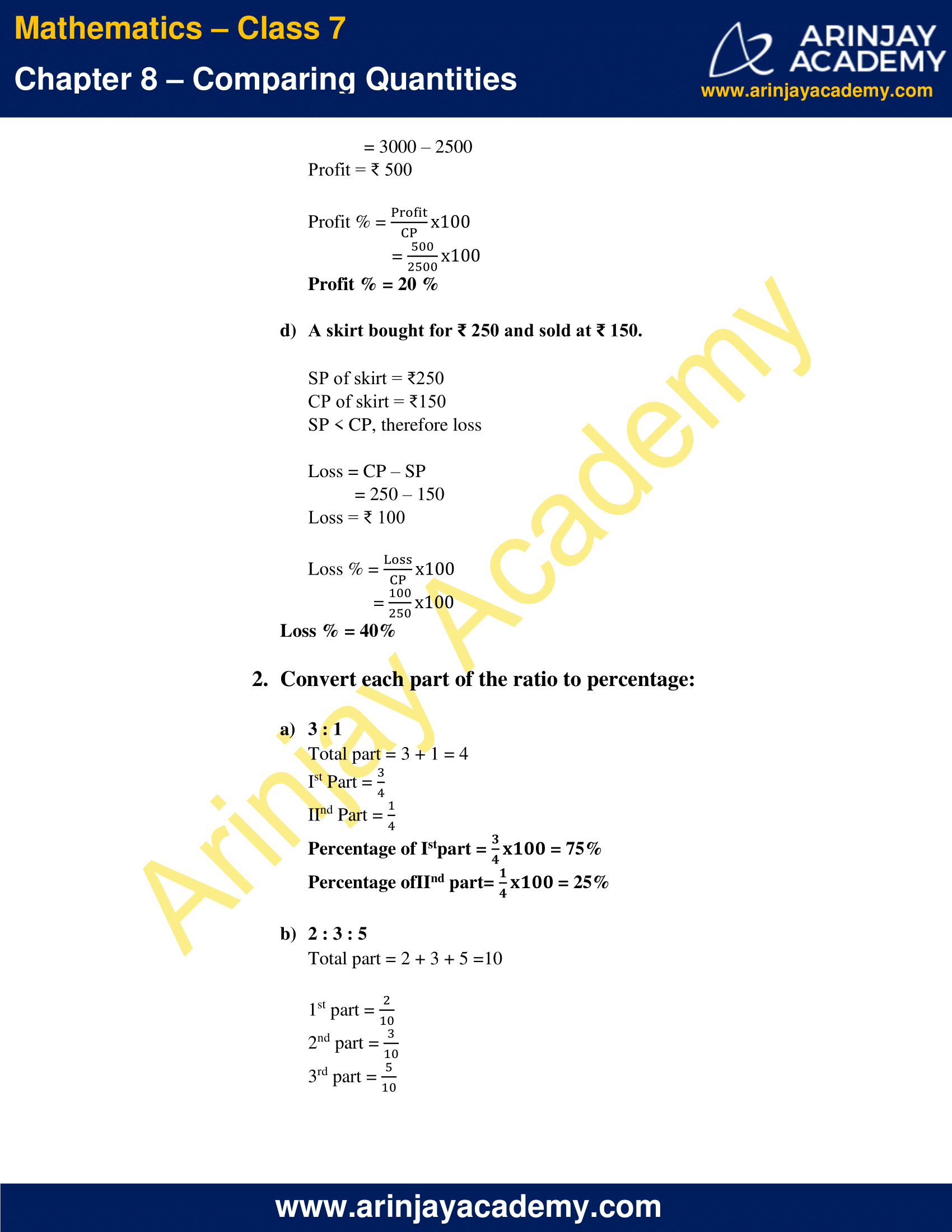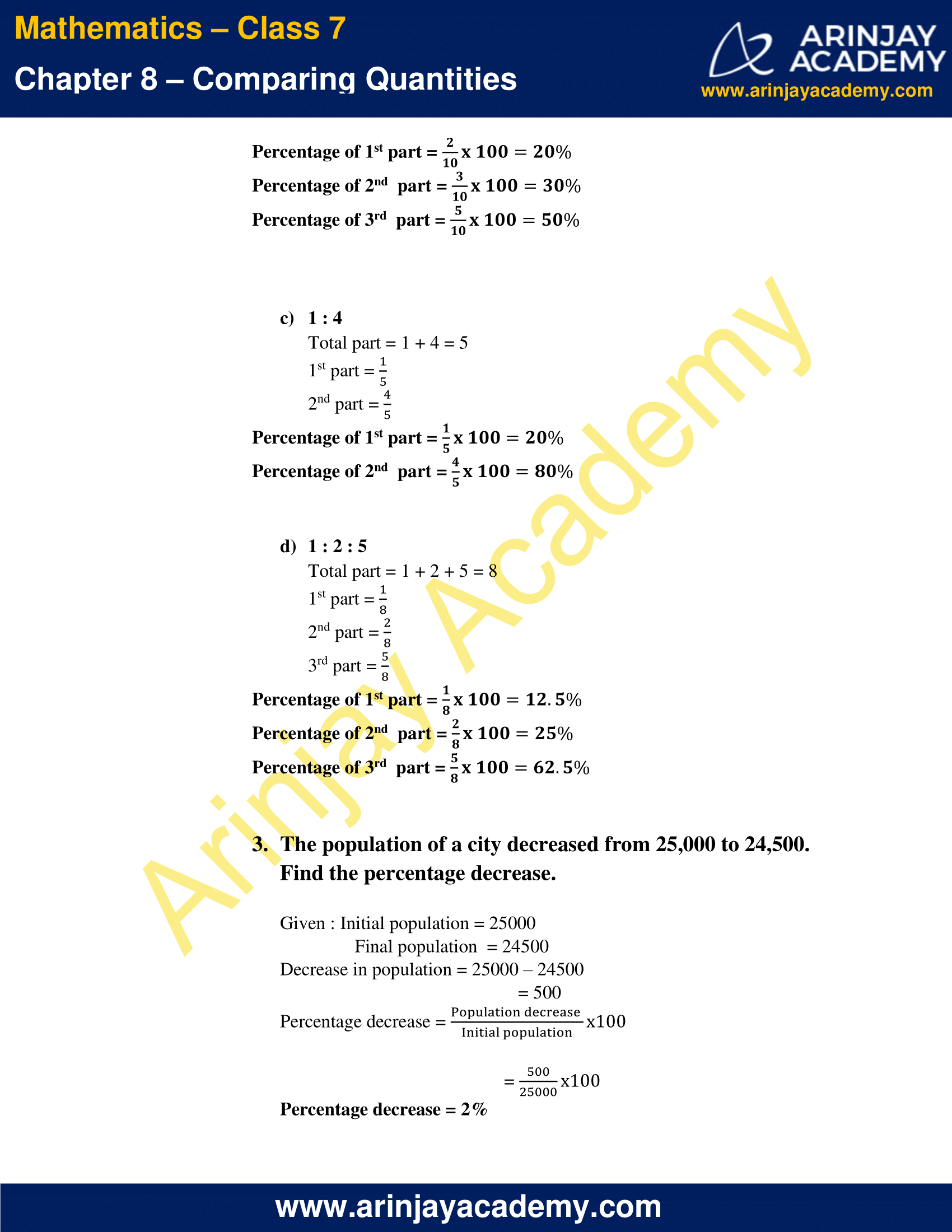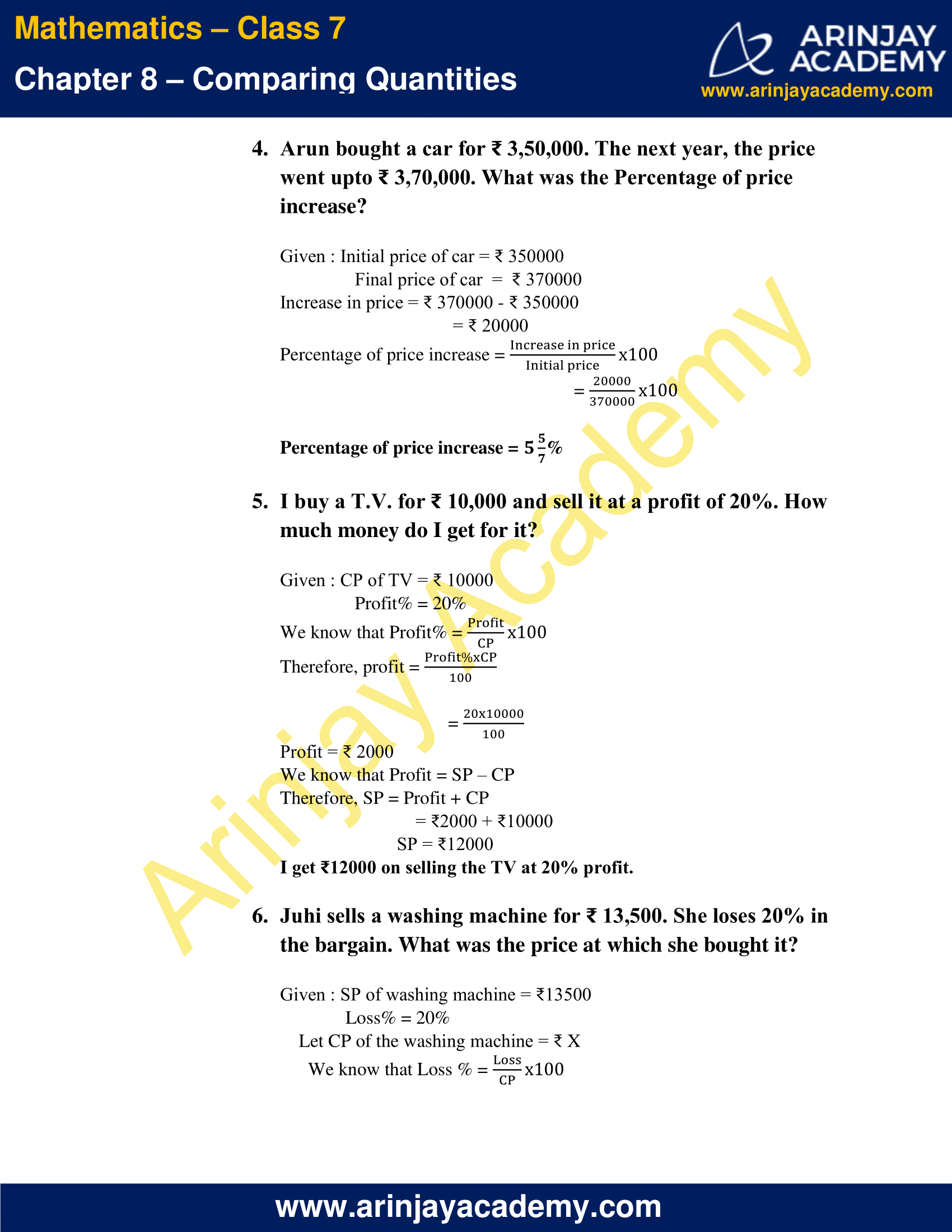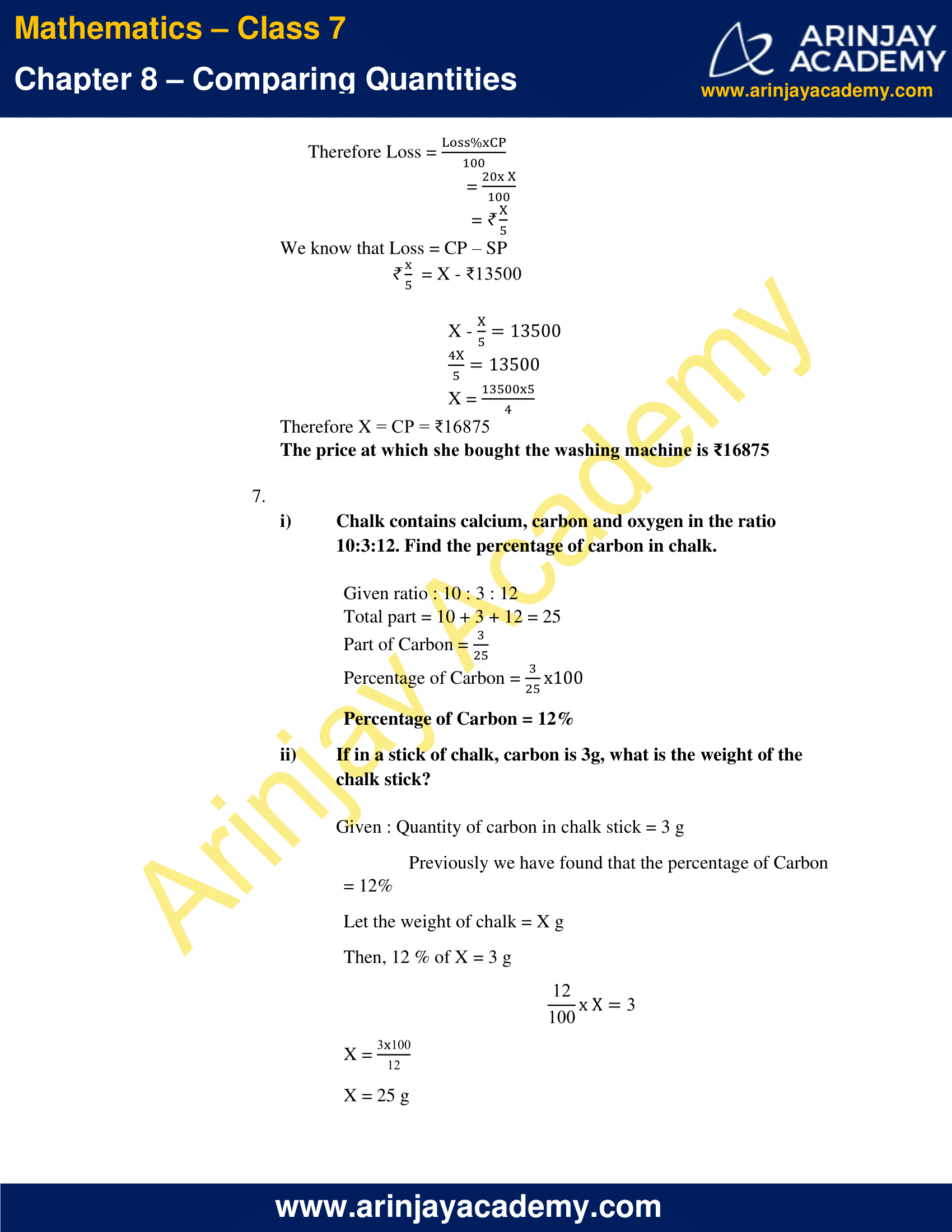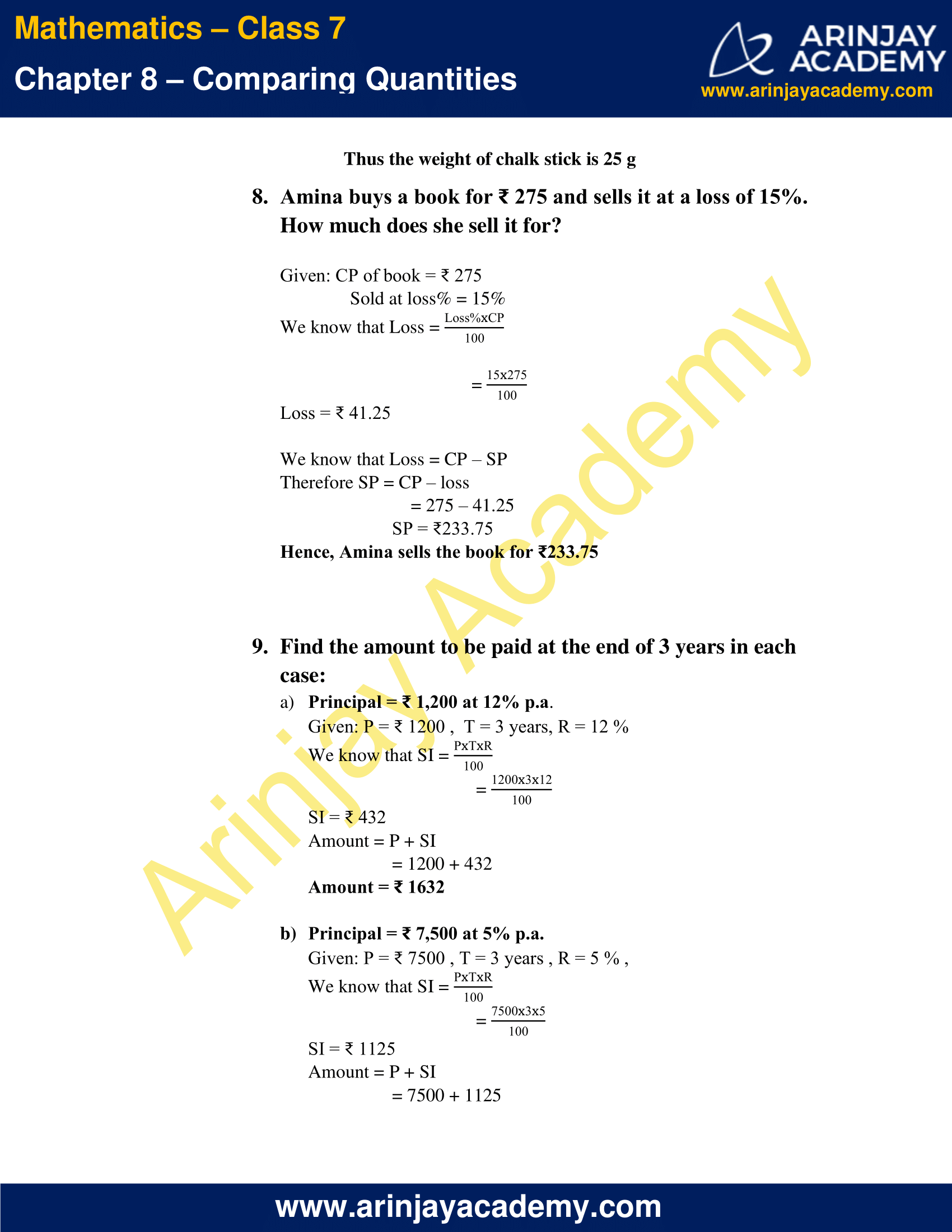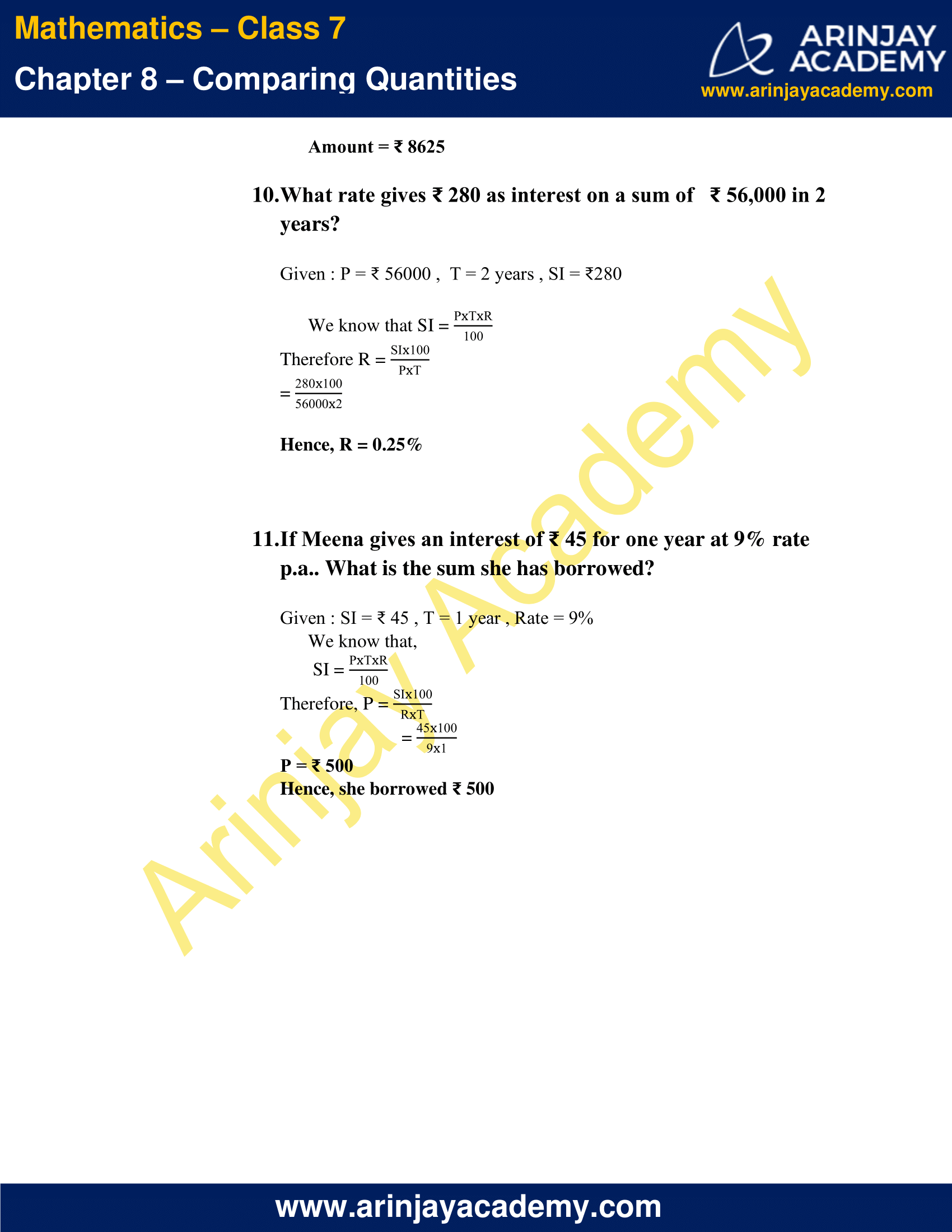### NCERT Solutions for Class 7 Maths Chapter 8 Exercise 8.1

1. Find the ratio of :

a) ₹5 to 50 paise

₹1 = 100 paise

₹5 = 100 x 5 = 500 paise

The required ratio is, 500/50 = 10/1 = 10 : 1

b) 15 kg to 210 g

1 kg = 1000 g

15 kg = 1000 g x 15  = 15000 g

The required ratio is, 15000/210  = 500/7 = 500 : 7

c) 9 m to 27 cm

1 m = 100 cm

9 m = 100 cm x 9 = 900cm

The required ratio, 900/27 = 100/3 = 100 : 3

d) 30 days to 36 hours

1 day = 24 hours

30 days = 24 hours x 30 = 720 hours

The required ratio is, 720/36 = 20/1 = 20 : 1

2. In a computer lab, there are 3 computers for every 6 students. How many computers will be needed for 24 students?

Given :  6 students need = 3 computers

1 student need =  3 x 1/6 = 1/2 computers

Therefore 24 students need  24 x 1/2 = 12 computers

3. Population of Rajasthan = 570 lakhs and population of UP = 1660 lakhs. Area of Rajasthan = 3 lakh km2 and area of UP = 2 lakh km2

i) How many people are there per km2 in both these States?

People present per km2 = Population/Area

In Rajasthan = (570 lakhs)/(3 lakhs per km²) = 190 lakhs people per km2

In UP = (1660 lakhs)/(2 lakhs per km²) = 830 lakhs people per  km2

ii) Which State is less populated?

From the above data, Rajasthan is less populated compared to UP.

### NCERT Solutions for Class 7 Maths Chapter 8 Exercise 8.2

1. Convert the given fractional numbers to per cents.

a) 1/8

= 1/8 x 100%

= 100/8 %

= 25/2 %

= 12 1⁄2 %

= 12.5%

b) 5/4

= 5/4 x 100%

= 125%

c) 3/40

= 3/40 x 100%

= 3/2 x 5%

= 15/2%

= 7 1⁄2 %

= 7.5%

d) 2/7

= 2/7 x 100%

= 200/7%

= 28 4⁄7 %%

2. Convert the given decimal fractions to per cents

a) 0.65

(65/100) x 100%

=65%

b) 2.1

(21/10) x 100%

=210%

c) 0.02

(2/100) x 100%

= 2%

d) 12.35

(1235/100) x 100%

= 1235%

3. Estimate what part of the figures is coloured and hence find the per cent which is coloured

i)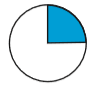The circle can be divided into 4 equal quadrants. In the given figure one quadrant is coloured.

i.e coloured part = 1/4

Therefore, per cent of coloured part = (1/4) x 100%

=25%

ii)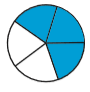In the given figure the circle is divided into 5 equal parts.

3 out of 5 parts are coloured.

i.e coloured part =3/5

Therefore, per cent of coloured part = (3/5) x 100%

= 60%

iii)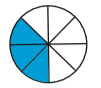In the given figure the circle is divided into 8 equal parts.

3 out of 8 parts are coloured.

i.e coloured part = 3/8

Therefore, per cent of coloured part = (3/8) x 100%

= 37.5%

4. Find :

a) 15% of 250

= (15/100) x 250

= 15 x 2.5

= 37.5%

b) 1% of 1 hour

1 hour = 60 minutes

= 60 x 60 seconds

1 hour = 3600 seconds

Therefore, 1% of 3600 seconds is

1/100 x 36000

=36 seconds

c) 20% of ₹2500

20/100 x 2500

=20 x 25

= ₹ 500

d) 75% of 1 kg

1 kg = 1000 g

Therefore 75% of 1000g is

75/100 x 1000

= 750 g

5. Find the whole quantity if:

a) 5% of it is 600

Let the whole value = a

(5/100) x a = 600

a = (600 x 100)/5

a = 12000

b) 12% of it is ₹1080

Let the whole value = ₹a

(12/100) x ₹a = ₹1080

a = (1080 x 100)/12

a = ₹ 9000

c) 40% of it is 500 km

Let the whole value = a km

(40/100) x a km = 500 km

a  = (500 x 100)/40

a  = 1250 km

d) 70% of it is 14 minutes

Let the whole value = a minutes

(7/100) x a minutes = 14 minutes

a  = (40 x 100)/70

a = 20 minutes

e) 8% of it is 40 litres

Let the whole value = a litres

(8/100) x a litres = 40 litres

a = (40 x 100)/8

a = 500 litres

6. Convert given percents to decimal fractions and also to fractions in simplest forms:

a) 25%

Fractions : 25/100

Simplest form : 1/4

Decimal form : 0.25

b) 150%

Fractions : 150/100

Simplest form : 3/2

Decimal form : 1.5

c) 20%

Fractions: 20/100

Simplest form : 1/5

Decimal form : 0.2

d) 5%

Fractions: 5/100

Simplest form : 1/20

Decimal form : 0.05

7. In a city, 30% are females, 40% are males and remaining are children. What per cent are children?

Given: Percentage of females = 30%

Percentage of males = 40%

Since remaining are children,

Percentage of children = 100% – (Percentage of females + males)

= 100% – (30 + 40)%

= 100% – 70%

Percentage of children = 30%

8. Out of 15,000 voters in a constituency, 60% voted. Find the percentage of voters who did not vote. Can you now find how many actually did not vote?

Given:  Total number of voters = 15000

Percentage of people who voted = 60%

Percentage of people who did not vote = 100% – 60%

= 40%

Therefore the number of people who did not vote = (40 x 100) x 15000

= 6000

Thus, 6000 people did not vote.

9. Meeta saves ₹ 4000 from her salary. If this is 10% of her salary. What is her salary?

Let Meeta’s salary = ₹ a

Given: (10/100) x a  = ₹ 4000

a = (4000 x 100)/10

a = ₹ 40000

Thus, Meeta’s salary is ₹ 40000

10. A local cricket team played 20 matches in one season. It won 25% of them. How many matches did they win?

Given: Total number of matches played = 20

Percentage of matches won = 25%

Number of matches won = (25/100) x 20

= 5

Thus, the number of matches won by the team is 5

### NCERT Solutions for Class 7 Maths Chapter 8 Exercise 8.3

1. Tell what is the profit or loss in the following transactions. Also find profit per cent or loss per cent in each case

a) Gardening shears bought for ₹ 250 and sold for ₹325.

CP of gardening shears = ₹ 250

SP of gardening shears = ₹ 325

SP > CP , therefore profit

Profit = SP – CP

= 325 – 250

Profit = ₹ 75

Profit % = (Profit/CP) x 100

= (75/250) x 100

Profit % = 30%

b) A refrigerator bought for ₹ 12,000 and sold at  ₹ 13,500

CP of refrigerator = ₹12000

SP of refrigerator = ₹13500

SP > CP, therefore profit

Profit = SP – CP

= 13500 – 12000

Profit = ₹ 1500

Profit % = Profit/CP

= 1500/1200

Profit% = 12.5 %

c) A cupboard bought for ₹ 2,500 and sold at ₹ 3,000.

CP of cupboard = ₹2500

SP of cupboard = ₹3000

SP > CP, therefore profit

Profit = SP – CP

= 3000 – 2500

Profit = ₹ 500

Profit % = (Profit/CP) x 100

= (500/2500) x 100

Profit % = 20 %

d) A skirt bought for ₹ 250 and sold at ₹ 150.

SP of skirt = ₹250

CP of skirt = ₹150

SP < CP, therefore loss

Loss = CP – SP

= 250 – 150

Loss = ₹ 100

Loss % = (Loss/CP) x 100

= (100/250) x 100

Loss % = 40%

2. Convert each part of the ratio to percentage:

a) 3 : 1

Total part = 3 + 1 = 4

Ist Part = 3/4

IInd Part = 1/4

Percentage of Istpart = (3/4) x 100 = 75%

Percentage of IInd part= (1/4) x 100 = 25%

b) 2 : 3 : 5

Total part = 2 + 3 + 5 =10

1st part = 2/10

2nd part = 3/10

3rd part = 5/10

Percentage of 1st part = (2/10) x 100 = 20%

Percentage of 2nd part = (3/10) x 100 = 30%

Percentage of 3rd part = (5/10) x 100 = 50%

c) 1 : 4

Total part = 1 + 4 = 5

1st part = 1/5

2nd part = 4/5

Percentage of 1st part = (1/5) x 100 = 20%

Percentage of 2nd part = (4/5) x 100 = 80%

d) 1 : 2 : 5

Total part = 1 + 2 + 5 = 8

1st part = 1/8

2nd part = 2/8

3rd part = 5/8

Percentage of 1st part = (1/8) x 100 = 12.5%

Percentage of 2nd part = (2/8) x 100 = 25%

Percentage of 3rd part = (5/8) x 100 = 62.5%

3. The population of a city decreased from 25,000 to 24,500. Find the percentage decrease.

Given : Initial population = 25000

Final population  = 24500

Decrease in population = 25000 – 24500

= 500

Percentage decrease = (Population decrease/Initial population) x 100

= 500/25000

Percentage decrease = 2%

4. Arun bought a car for ₹ 3,50,000. The next year, the price went upto ₹ 3,70,000. What was the Percentage of price increase?

Given : Initial price of car = ₹ 350000

Final price of car  =  ₹ 370000

Increase in price = ₹ 370000 – ₹ 350000

= ₹ 20000

Percentage of price increase = (Increase in price/Initial price) x 100

= 20000/370000

Percentage of price increase = 5 5⁄7 %

5. I buy a T.V. for ₹ 10,000 and sell it at a profit of 20%. How much money do I get for it?

Given : CP of TV = ₹ 10000

Profit% = 20%

We know that Profit% = (Profit/CP) x 100

Therefore, profit = Profit% x  CP/100

= (20 x 10000)/100

Profit = ₹ 2000

We know that Profit = SP – CP

Therefore, SP = Profit + CP

= ₹2000 + ₹10000

SP = ₹12000

I get ₹12000 on selling the TV at 20% profit.

6. Juhi sells a washing machine for ₹ 13,500. She loses 20% in the bargain. What was the price at which she bought it?

Given : SP of washing machine = ₹13500

Loss% = 20%

Let CP of the washing machine = ₹ X

We know that Loss % = (Loss/CP) x 100

Therefore Loss = Loss% x  CP/ 100

= (20 x X)/100

= ₹(X/5)

We know that Loss = CP – SP

₹ (X/5) = X – ₹13500

X – (X/5) = 13500

(4X/5) = 13500

X = (13500 x X)/4

Therefore X = CP = ₹16875

The price at which she bought the washing machine is ₹16875

7. (i) Chalk contains calcium, carbon and oxygen in the ratio 10:3:12. Find the percentage of carbon in chalk.

Given ratio : 10 : 3 : 12

Total part = 10 + 3 + 12 = 25

Part of Carbon =3/25

Percentage of Carbon = (3/25) x 100

Percentage of Carbon = 12%

(ii) If in a stick of chalk, carbon is 3g, what is the weight of the chalk stick?

Given : Quantity of carbon in chalk stick = 3 g

Previously we have found that the percentage of Carbon = 12%

Let the weight of chalk = X g

Then, 12 % of X = 3 g

12/100 x X = 3

X = (3 x 100)/12

X = 25 g

Thus the weight of chalk stick is 25 g

8. Amina buys a book for ₹ 275 and sells it at a loss of 15%. How much does she sell it for

Given: CP of book = ₹ 275

Sold at loss% = 15%

We know that Loss = Loss% x  CP/ 100

= (15 x 275)/100

Loss = ₹ 41.25

We know that Loss = CP – SP

Therefore SP = CP – loss

= 275 – 41.25

SP = ₹233.75

Hence, Amina sells the book for ₹233.75

9. Find the amount to be paid at the end of 3 years in each case:

a) Principal = ₹ 1,200 at 12% p.a.

Given: P = ₹ 1200 ,  T = 3 years, R = 12 %

We know that SI = (P x T x R)/100

= (1200 x 3 x 12)/100

SI = ₹ 432

Amount = P + SI

= 1200 + 432

Amount = ₹ 1632

b) Principal = ₹ 7,500 at 5% p.a.

Given: P = ₹ 7500 , T = 3 years , R = 5 % ,

We know that SI = (P x T x R)/100

= (7500 x 3 x 5)/100

SI = ₹ 1125

Amount = P + SI

= 7500 + 1125

Amount = ₹ 8625

10. What rate gives ₹ 280 as interest on a sum of ₹ 56,000 in 2 years?

Given : P = ₹ 56000 ,  T = 2 years , SI = ₹280

We know that SI = (P x T x R)/100

Therefore R = (SI x 100)/(P x T)

= (280 x 100)/(56000 x 2)

Hence, R = 0.25%

11. If Meena gives an interest of ₹ 45 for one year at 9% rate p.a.. What is the sum she has borrowed?

Given : SI = ₹ 45 , T = 1 year , Rate = 9%

We know that,

SI = (SI x 100)/(P x T)

Therefore, P = (SI x 100)/(R x T)

= (45 x 100)/(9 x 1)

P = ₹ 500

Hence, she borrowed ₹ 500

With this we come to the end of NCERT Solutions for Class 7 Maths Chapter 8 Comparing Quantities. We hope these helped you study your subject.

Download NCERT Solutions for Class 7 Maths Chapter 8 – Comparing Quantities

### 1 thought on “NCERT Solutions for Class 7 Maths Chapter 8 – Comparing Quantities”

1.I have amazingly found this academy my name is also Arinjay only.

Reply